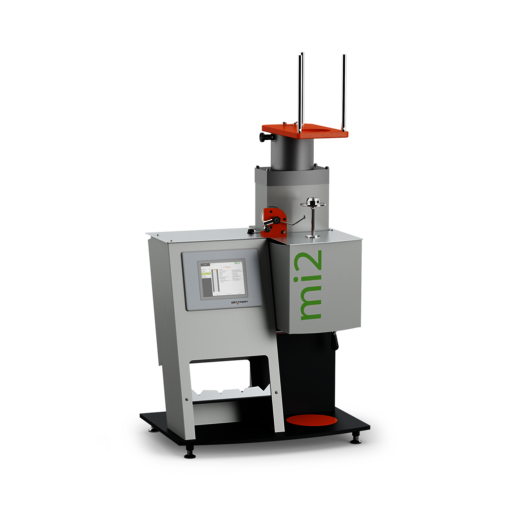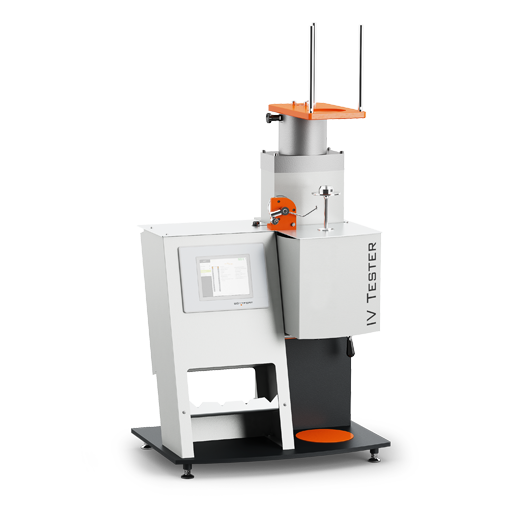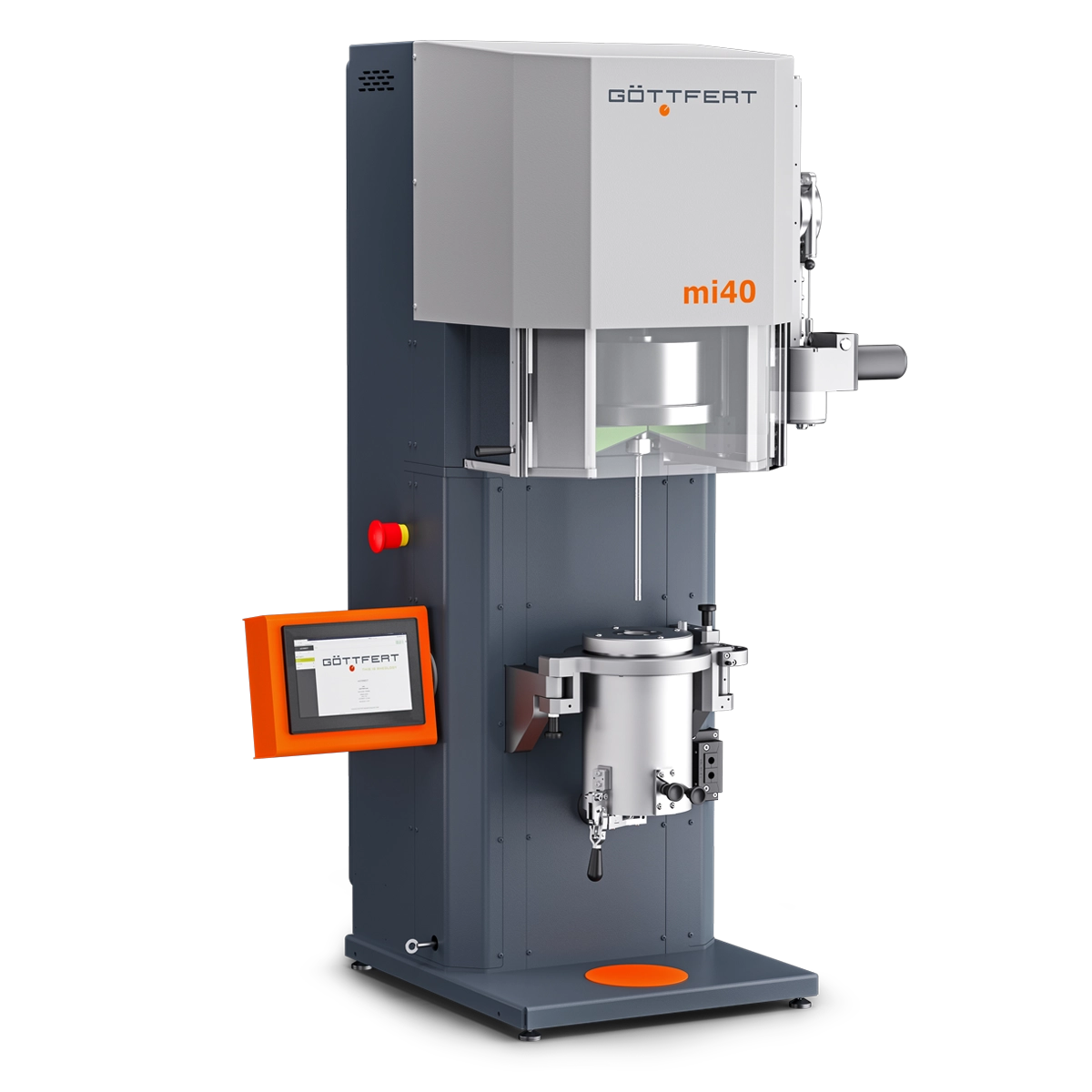## The Detection of the Intrinsic Viscosity (IV)

To characterize the average molecular weight on Polyester (PET) and Polyamid (PA) based plastics very often the solvent viscosity is being used, also called intrinsic viscosity. This value is very time-consuming to determine and the incoming solvents might be harmful while handling as well as circumstantial in the disposal. In many cases this detected value is afflicted with a wide error spread of the results. Automatic measuring devices to determine the intrinsic viscosity might be very complex.

By contrast to this procedure the melt index or also the melt viscosity, determined with Melt Index Devices, also called Plastometer, as well as Laboratory or Online Capillary Rheometers, can be found out in an easy and fast way. The user friendly handling is uncomplicated. Details to the specific devices can be drawn from our respective product descriptions.

The determination of the intrinsic viscosity and the display of the IV value comes automatically in all Goettfert test devices or after selection in the specific software.
Please see the technical documentation / operating manual / software documentation of the respective device for more handling details.

Application

Characterization of the average molecular weight

Calculation of the Intrinsic Viscosity

The determination of the intrinsic viscosity is performed through a correlation relationship. This is being defined by a one-time detected and representative data set of a product group out of measured melt index or melt viscosity values and measured intrinsic viscosities (IV). The data set should be detected for minimum 3 pair of variates IV values and melt index or melt viscosities. With a higher number of pair of variates a higher accuracy for the IV value via the correlation relationship can be reached. On time determined this function can be used also for other materials with similar filler content.

The following 2 diagrams show as an example for PET a very good correlation between melt index and IV value as well as melt viscosity and IV value:

For the correlation relationship between intrinsic viscosity and melt index or melt viscosity the following combinations are valid, which describe finally the temperature dependences of the melt index or the melt viscosities.

Via the average value of the MFR or MVR the intrinsic viscosity IVRheo is being calculated as follows: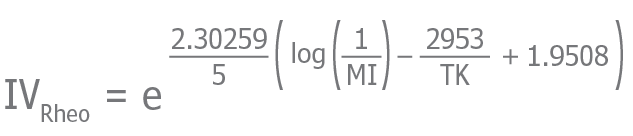Equation 1

Also comparable with the calculated or measured melt viscosity η
(here at the melt viscosity a preferable way is to use the zero shear viscosity η0 ;
to determine the zero shear viscosity models like Yasuda or Carreau-Winter can be used. These models are integrated in the evaluation software “WinRheo II”).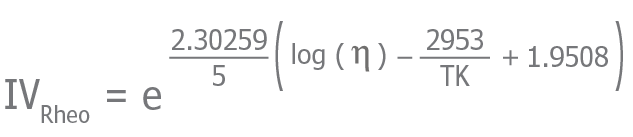Equation 2

Here are:

TK      =  Test temperature in °K
A        =  Axis intercept (constant)
MI      =  Average value of MFR or MVR
η        =  Viscosity

The result of this is a correlation between the measured and the calculated intrinsic viscosities IV and IVRheo (see diagram 3). This correlation relationship is a linear equation with 2 constants A and B.Equation 3

The constants A and B must be determined first, to proceed the calculation. The constants are only valid for one test weight or one shear rate under the precondition, that the measurement procedure of the intrinsic viscosity has been performed always in the same way. After the constants were being found out, these values are saved in the device or in the PC software.

The calculation of the intrinsic viscosity has been proceed after the measurement. At first the IVRheo is being calculated with the measured MI average value or the melt viscosity according to equation 1 or 2. Afterwards the IV is being detected out of the calculated IVRheo and the known coefficients A and B according to equation No. 3.

One IV value is being calculated out of the average value of the MI measurement or out of the viscosity for each weight or shear rate. We recommend to determine the constants for each product spectrum separately new because the IV value can depend from the measurement method and the used solvent in a wide range.

Determination of the constants A and B

To determine the constants A and B a minimum number of 3 pair of variates from the measured melt index or the melt viscosity and measured IV viscosity are required.

The following example has been performed with 7 meltindex measurements, to improve the accuracy of the approximation (see diagram No. 1):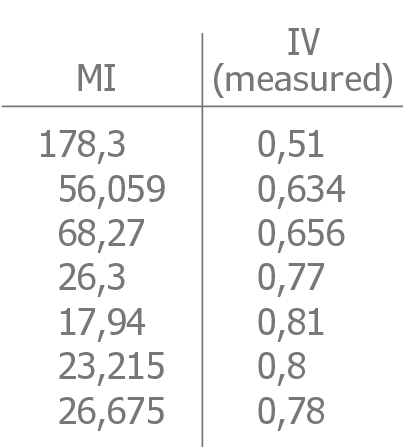The value IVRheo has been detected via the equation No. 1 out of the melt index value.
Getting the following table: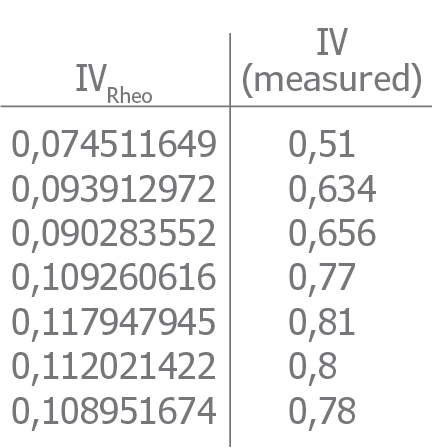The values are being applied graphically to determine the coefficients A and B. In this case the points needs to be approximated in a linear way.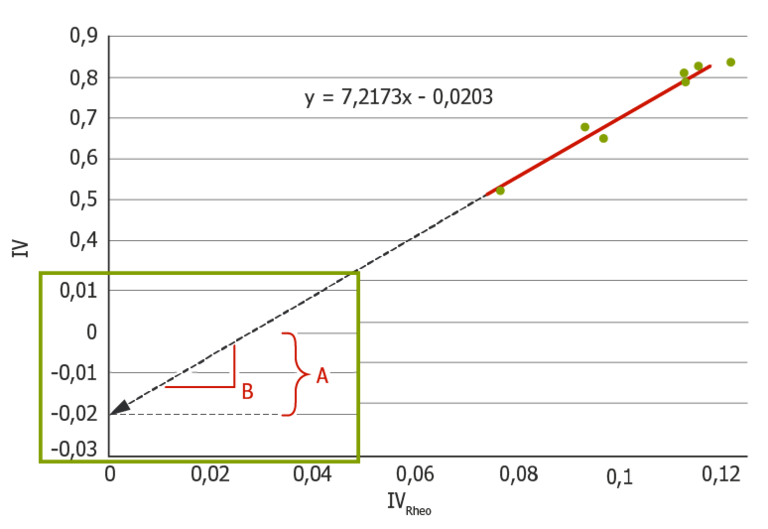Diagram 3: Correlation between the measured (IV) and the calculated IVRheo intrinsic viscosities

Out of the positioning of the measuring points and the linear approximation the constants A and B are A = -0,0203 and B = 7,2173.

## Products